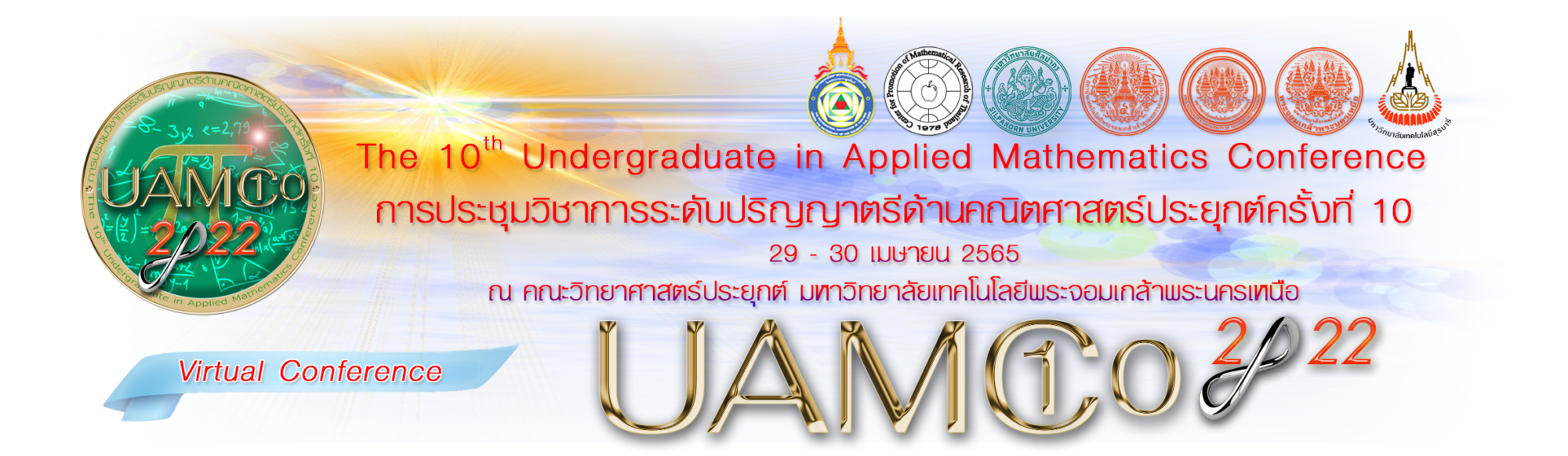Search for:

กลุ่มที่ 1     Numerical Methods and Applications

Numerical approximation and computation, error analysis and interval analysis, acceleration of convergence, mathematical programming, numerical optimization and variational techniques, approximation methods and numerical treatment of dynamical systems, integral transforms, numerical methods in Fourier analysis, computer aspects of numerical algorithms, applications of numerical methods to physics and engineering problems.

กลุ่มที่ 2     Mathematical Modelling

Analysis of mathematical models, validation and verification of mathematical models, topological dynamics, Hamiltonian and Lagrangian systems, dynamical analysis of models, stability analysis, local and nonlocal bifurcation theory, controllability and observability, system structure mathematical biology, genetics and population dynamics, calculus of variations and optimal control, optimization models, stochastic and probability models.

กลุ่มที่ 3     Mathematics for Industry

Industrial mathematics, operations research, logistics and supply chain management, optimization problems in industry, engineering modeling and simulations, robust geometric modeling, mathematical challenges from industry, analytical and problem-solving on industrial issue, applications in industry and business. calculus of variations and optimal control, optimization models. mathematics of industrial security.

กลุ่มที่ 4     Mathematics for Finance and Insurance

Financial mathematics, actuarial mathematics, mathematics for economics, business mathematics, risk analysis and management, investment mathematics, quantitative analysis in finance, financial analytics, interest theory, fuzzy logic for business and finance, financial derivatives, econometrics, engineering economy, analytical and problem-solving on business and management, mathematical models in finance and insurance.

กลุ่มที่ 5     Computational Mathematics

Computer system organization, theory of data, big data analysis and data mining, theory of computation and programming, discrete mathematics in relation to computer science, computability and recursion theory, artificial intelligence, evolutionary computation, computing methodologies and applications, algorithms in combinatorics and graph theory, communication and information, theory of error-correcting codes and error-detecting codes, fuzzy sets and logic, mathematics for computer graphics, image processing.

กลุ่มที่ 6     Algebra and Analysis with Applications

Algebra, linear algebra, semigroups, universal algebra, algebraic structure, number theory, analytical number theory, coding theory, graph theory, combinatorics, algebraic geometry, field theory and polynomial, set theory, logic and applications of logic, analysis, functional analysis, complex analysis, theory of differential equations, applied analysis, topology, differential geometry, differential topology, calculus theory, fractional derivative and integral, topological group, Lie group, Lie algebra, group analysis, Fourier analysis, wavelet, game theory.

กลุ่มที่ 7     Statistics and Data Analytics

Foundational topics in statistics, statistical sampling theory, statistical distribution theory, survival analysis and censored data, parametric and nonparametric inferences, sequential statistical methods, design of statistical experiments, multivariate analysis, big data analysis, data analytics and visualization, data mining, machine learning, database management, electronic data processing, algorithms for data analysis.

กลุ่มที่ 8     Mathematics for Sustainable Development Goals

Mathematical modeling as a tool for sustainable development, philosophy of mathematics education for sustainable development,  mathematics of planet earth, mathematical and statistical Challenges for sustainability, sustainable management of natural resources, assessment and simulation tools for sustainable energy systems, mathematics of energy and climate change, social multi-criteria evaluation for sustainable economy, quantitative methods and mathematical techniques for environmental performance evaluation.